## Introduction

This vignette illustrates how to get started with cellmigRation, an R library aimed at analyzing cell movements over time using multi-stack tiff images of fluorescent cells.

The software includes two modules:

• Module 1: data import and pre-precessing. This module includes a series of functions to import tiff images, remove noise/background and detect cell/particles, (optional) automatically estimate optimal analytic parameters, compute cell tracks (movements) and basic stats. The first module is largely based on the FastTracks software written in Matlab by Brian DuChez (FastTracks, https://www.mathworks.com/matlabcentral/fileexchange/60349-fasttracks, MATLAB Central File Exchange).

• Module 2: advanced analyses and visualization. The second module includes a series of functions to compute advanced metrics/stats, exporting, automatically built visualizations, and generate interactive/3D plots.

## Summary

This vignette guides the user through package installation, tiff file import, cell tracking, and a series of downstream analyses.

• Package installation

• Module 1

• Importing TIFF files

• Optimizing Tracking Params

• Tracking Cell Movements

• Basic migration stats

• Basic visualizations

• Aggregate Cell Tracks

• Module 2

• Import and Pre-process Cell Tracks

• Plotting tracks (2D and 3D)

• Deep Trajectory Analysis

• Final Results

• Principal Component Analysis (PCA) and Cell Clustering

### Notes and Acknowledgmenets

Damiano Fantini (Northwestern University, Chicago, IL, USA); Salim Ghannoum (University of Oslo, Oslo, Norway)

### Reproducibility

For reproducibility of the output on this document, please run the following command in your R session before proceeding:

``set.seed(1234)``

## Installation

The package is currently available on Bioconductor. It can be installed using the following command:

``````if(!requireNamespace("BiocManager", quietly = TRUE))
install.packages("BiocManager")
BiocManager::install("cellmigRation")``````

## cellmigRation Pipeline

#### Required libraries

For this demo, the following libraries have to be loaded.

``````library(cellmigRation)
library(dplyr)
library(ggplot2)
library(kableExtra)``````

### Module 1

#### Importing TIFF files

In this vignette, we are going to analyze three images with the aim of illustrating the functions included in cellmigRation. The original TIFF files are available at the following URLs:

Note. TIFF files can be imported using the `LoadTiff()` function. This function includes a series of (optional) arguments to attach meta-information to a TIFF image, for example the `experiment` and `condition` arguments. Imported numeric images are stored as a trackedCells-class object.

Three sample `trackedCells` objects (imported from the corresponding TIFF files) are available as a list in the `cellmigRation` package (`ThreeConditions` object). These will be used for illustrating the functions of our package in this vignette.

``````# load data
data(ThreeConditions)

# An S4 trackedCells object
ThreeConditions[]
#>  ~~~ An S4 trackedCells object ~~~
#>
#>       + Num of images: 7
#>       + Optimized Params: No
#>       + Run w/ Custom Params: No
#>       + Cells Tracked: No
#>       + Stats Computed: No``````

#### Optimizing Tracking Params

This is an optional yet recommended step. Detecting fluorescent cells requires defining a series of parameters to maximize signal to noise ratio. Specifically,

• diameter: size corresponding to the largest diameter of a cell (expressed in pixels). Ideally, we want to set this parameter to a value large enough to capture all cells (even the large ones), but small enough to exclude aggregates or large background particles (artifacts, bubbles)

• lnoise: size corresponding to the smalles diameter of a cell (expressed in pixels). Ideally, we want to set this parameter to a value small enough to capture all cells (even the small ones), but large enough to exclude small background particles (artifacts, debris)

• threshold: signal level used as background threshold. Signal smaller than threshold is set to zero

If the values of these arguments are known, you can skip this step. Alternatively, if you want to test a specific range of these values, you can run `OptimizeParams()` manually specifying the ranges to be tested. By default, the function determines automatically a reasonable range of values to be tested for each argument based on the empirical distribution of signal and sizes of particles detected in the frame with median signal from a TIFF stack. This operation supports parallelization (recommended: parallelize by setting the `threads` argument to a value bigger than 1).

Note: the user may request to visualize a plot. The output plot shows how many cells were detected for each combination of parameter values. By default, the pick #1 is selected for the downstream steps.

Note 2: for larger datasets, the user may wish to set the `threads` argument below to a larger integer in order to benefit from paralellized operations. A theoretical upper bound to this argument would be the number of threads in your CPU—which you can check with `parallel::detectCores()`—, but it is considered good practice to leave at least one thread for other system operations.

``````# Optimize parameters using 1 core
x1 <- OptimizeParams(
ThreeConditions\$ctrl01, threads = 1, lnoise_range = c(5, 12),
diameter_range = c(16, 22), threshold_range = c(5, 15, 30),
verbose = FALSE, plot = TRUE)``````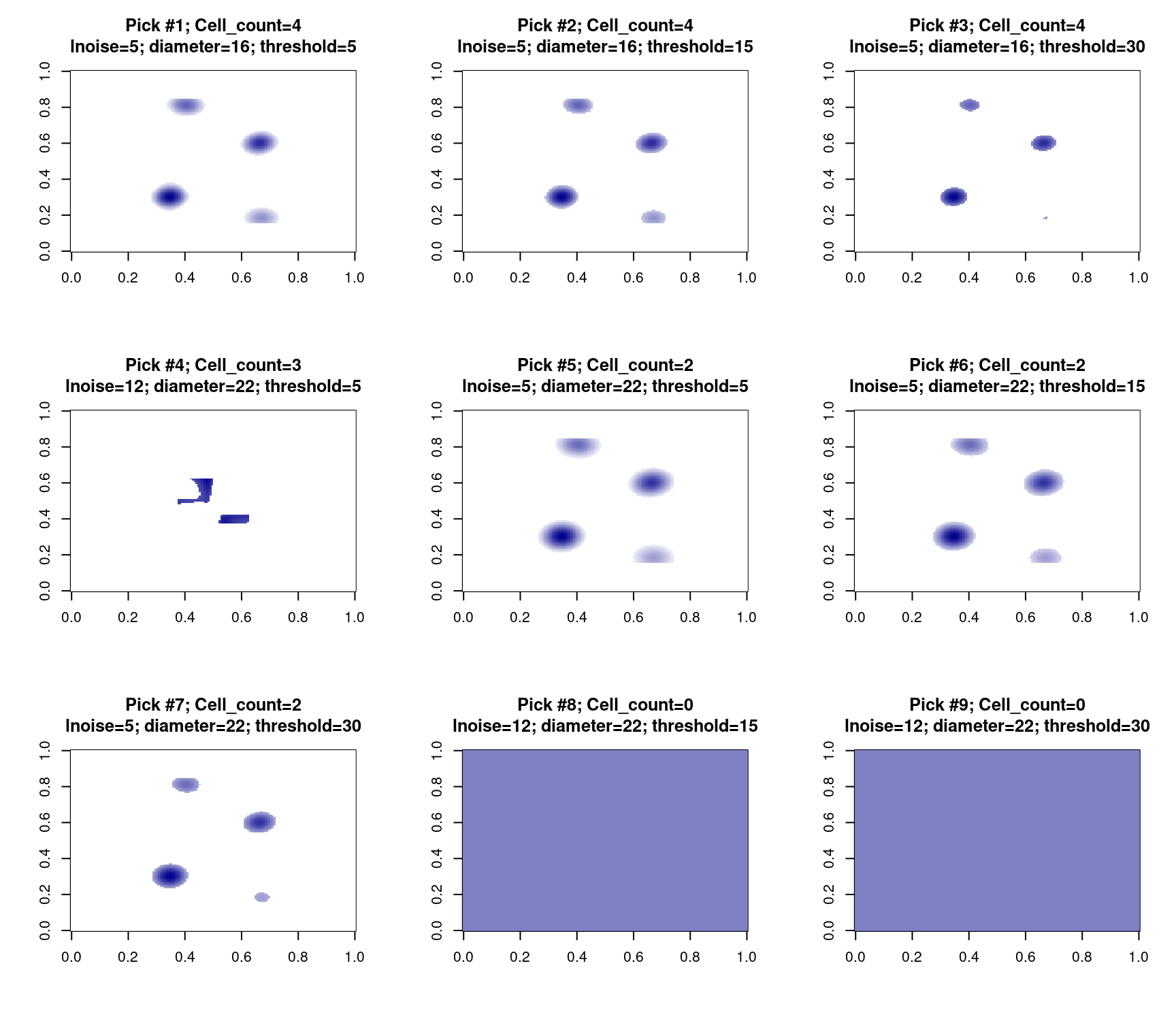Note 3: the `getOptimizedParams()` is a getter function to obtain the values of each optimized parameter.

``````# obtain optimized params
getOptimizedParams(x1)\$auto_params
#> \$lnoise
#>  5
#>
#> \$diameter
#>  16
#>
#> \$threshold
#>  5``````

#### Tracking Cell Movements

The central step of Module 1 is tracking cell movements across all frames of a multi-stack image (where each stack was acquired at a different time). This operation is carried out via the `CellTracker()` function, which performs two tasks: i) identify all cells in each frame of the image; ii) map cells across all image frames, identify cell movements and return cell tracks. This operation supports parallelization. This function requires three parameters to be set: `lnoise`, `diameter`, and `threshold`. These parameters can be set manually or automatically:

• rely on the optimized params estimated using `OptimizeParams()`

• rely on the optimized params estimated for a different `trackedCells` object; using `OptimizeParams()`; see the `import_optiParam_from` argument

• the user can manually specify the parameter values; note that user-specified parameters will overwrite automatically-optimized values

Note 1: the user may request to visualize a plot for each frame being processed. The output plot shows cells that were detected for each combination of parameter values.

Note 2: it is possible to only include cells that were detected in at least a minimum number of frames by setting the `min_frames_per_cell` argument. If so, cells detected in a small number of frames will be removed from the output.

Note 3: the user may parallelize (recommended when possible) this step by setting the `threads` argument to a value bigger than 1.

``````# Track cell movements using optimized params
x1 <- CellTracker(
tc_obj = x1, min_frames_per_cell = 3, threads = 1, verbose = TRUE)

# Track cell movements using params from a different object
x2 <- CellTracker(
ThreeConditions\$ctrl02, import_optiParam_from = x1,
min_frames_per_cell = 3, threads = 1)``````
``````# Track cell movements using CUSTOM params, show plots
x3 <- CellTracker(
tc_obj = ThreeConditions\$drug01,
lnoise = 5, diameter = 22, threshold = 6,
threads = 1, maxDisp = 10,
show_plots = TRUE)``````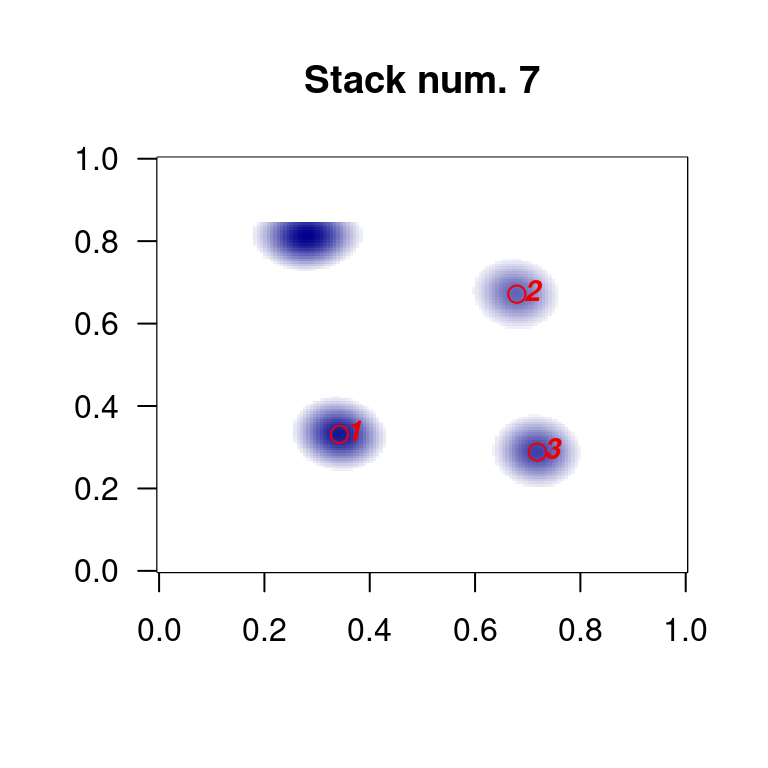It is possible to retrieve the output data.frame including information about cell movements (cell tracks) using the `getTracks()` getter function.

``````# Get tracks and show header
trk1 <- cellmigRation::getTracks(x1)
head(trk1) %>% kable() %>% kable_styling(bootstrap_options = 'striped')``````
cell.ID X Y frame.ID
1 42.38249 37.14955 1
1 50.38249 41.14955 2
1 57.38249 35.14955 3
1 65.38798 31.75083 4
1 72.38250 37.14957 5
1 83.38800 31.75101 6

#### Basic migration stats

For compatibility and portability reasons, Module 1 includes a function to compute the same basic metrics/stats as in the FastTracks Matlab software by Brian DuChez. This step is performed via the `ComputeTracksStats()` function. The results can be extracted from a `trackedCells` object via dedicated getter functions: `getPopulationStats()` and `getCellsStats()`. Note however that more advanced stats are computed using functions included in the second module of `cellmigRation`.

``````# Basic migration stats can be computed similar to the fastTracks software
x1 <- ComputeTracksStats(
x1, time_between_frames = 10, resolution_pixel_per_micron = 1.24)
x2 <- ComputeTracksStats(
x2, time_between_frames = 10, resolution_pixel_per_micron = 1.24)
x3 <- ComputeTracksStats(
x3, time_between_frames = 10, resolution_pixel_per_micron = 1.24)

# Fetch population stats and attach a column with a sample label
stats.x1 <- cellmigRation::getCellsStats(x1) %>%
mutate(Condition = "CTRL1")
stats.x2 <- cellmigRation::getCellsStats(x2) %>%
mutate(Condition = "CTRL2")
stats.x3 <- cellmigRation::getCellsStats(x3) %>%
mutate(Condition = "DRUG1")``````
``````stats.x1 %>%
dplyr::select(
c("Condition", "Cell_Number", "Speed", "Distance", "Frames")) %>%
kable() %>% kable_styling(bootstrap_options = 'striped')``````
Condition Cell_Number Speed Distance Frames
CTRL1 1 1.1812662 70.87597 6
CTRL1 2 1.3511152 81.06691 6
CTRL1 3 0.9840036 59.04022 6
CTRL1 4 1.1166609 66.99965 6
``````# Run a simple Speed test
sp.df <- rbind(
stats.x1 %>% dplyr::select(c("Condition", "Speed")),
stats.x2 %>% dplyr::select(c("Condition", "Speed")),
stats.x3 %>% dplyr::select(c("Condition", "Speed"))
)

vp1 <- ggplot(sp.df, aes(x=Condition, y = Speed, fill = Condition)) +
geom_violin(trim = FALSE) +
scale_fill_manual(values = c("#b8e186", "#86e1b7", "#b54eb4")) +
geom_boxplot(width = 0.12, fill = "#d9d9d9")

print(vp1)``````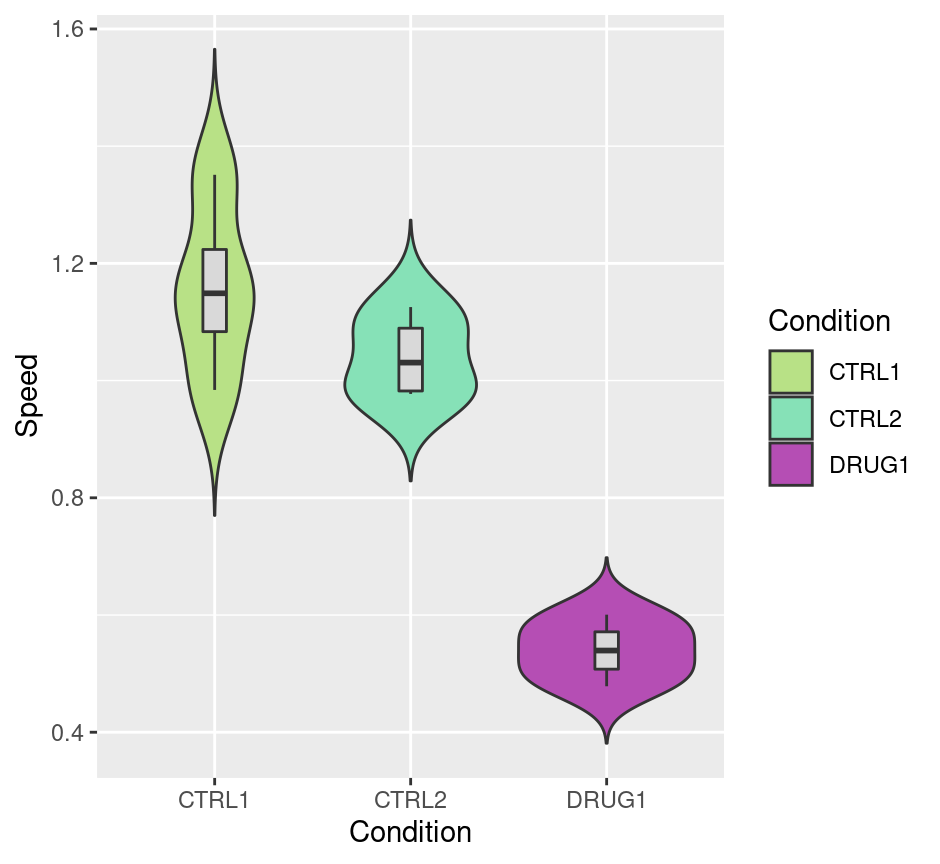``````# Run a t-test:
sp.lst <- with( sp.df, split(Speed, f = Condition))
t.test(sp.lst\$CTRL1, sp.lst\$DRUG1, paired = FALSE, var.equal = FALSE)
#>
#>  Welch Two
#>  Sample
#>  t-test
#>
#> data:  sp.lst\$CTRL1 and sp.lst\$DRUG1
#> t = 7.6628,
#> df =
#> 3.7144,
#> p-value =
#> 0.002093
#> alternative hypothesis: true difference in means is not equal to 0
#> 95 percent confidence interval:
#>  0.3876210 0.8499647
#> sample estimates:
#> mean of x
#> 1.1582615
#> mean of y
#> 0.5394686``````

#### Basic Visualizations

Two basic visualization functions are included in Module 1, and allow visualization of cells detected in a frame of interest, and tracks originating at a frame of interest. These functions are included in Module 1 (and not Module 2) since they take a `trackedCells`-class object as input.

``````# Visualize cells in a frame of interest
cellmigRation::VisualizeStackCentroids(x1, stack = 1)``````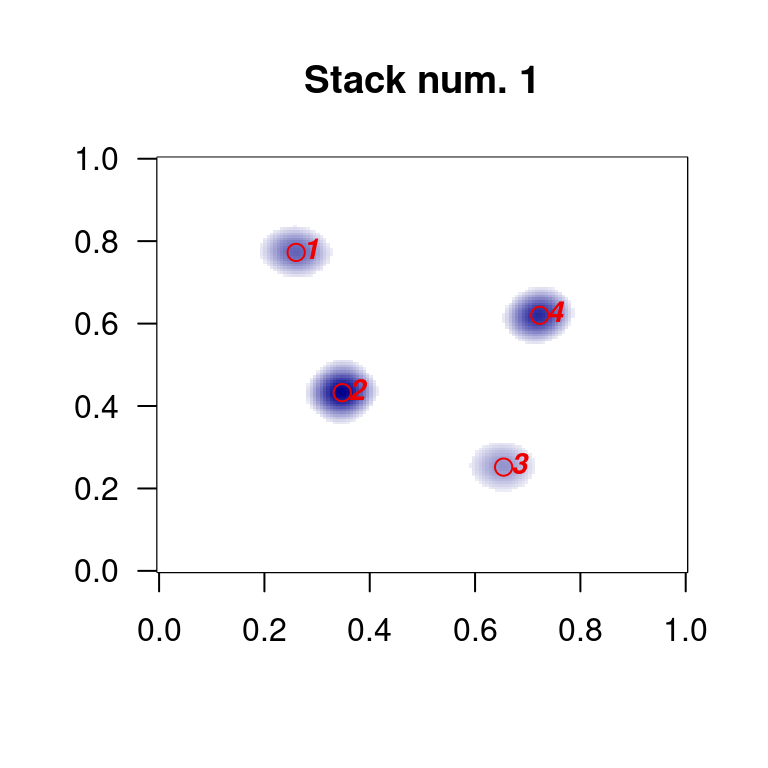``````# Visualize tracks of cells originating at a frame of interest
par(mfrow = c(1, 3))
cellmigRation::visualizeCellTracks(x1, stack = 1, main = "tracks from CTRL1")
cellmigRation::visualizeCellTracks(x2, stack = 1, main = "tracks from CTRL2")
cellmigRation::visualizeCellTracks(x3, stack = 1, main = "tracks from DRUG1")``````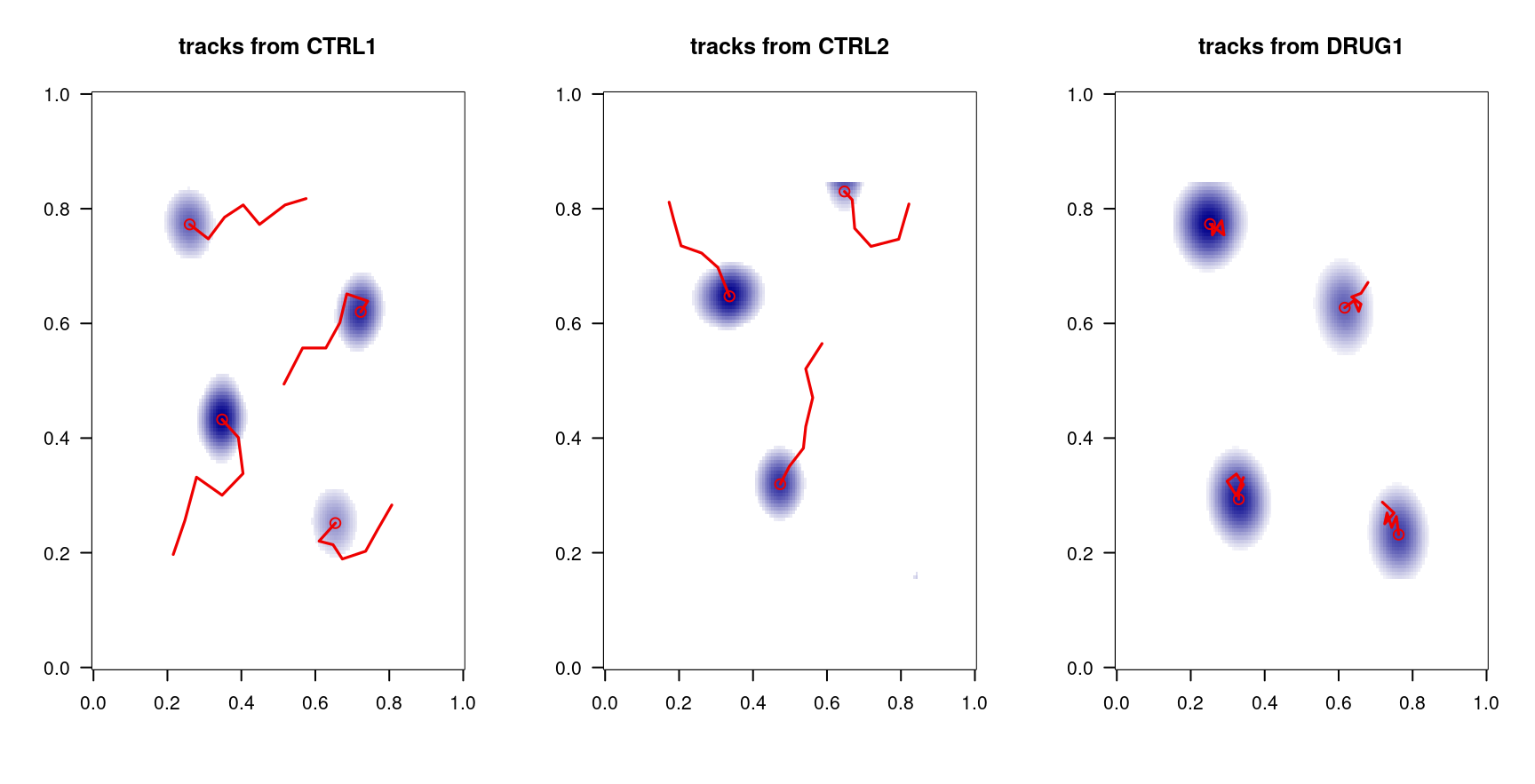#### Aggregate Cell Tracks

Cell tracks from multiple TIFF images can be aggregated together. All tracks form the different experiments/images are returned in a large data.frame. A new unique ID is assigned to specifically identify each cell track from each image/experiment. Different `trackedCells` objects can be merged together based on the corresponding TIFF filename (default), or one of the meta-information included in the object(s).

Note 1: the data.frame returned by `aggregateTrackedCells()` has a structure that aligns to the output of the `getTracks()` function when the `attach_meta` argument is set to TRUE.

Note 2: the data.frame returned by `aggregateTrackedCells()` (or by `getTracks()` with the `attach_meta` argument set to TRUE) is the input of the `CellMig()` function, and is the first step of Module 2.

Note 3: it is recommended to aggregate experiments/tiff files corresponding to the same condition (as shown below: for example, all replicates of the control cells) However, it is also possible to mix and match multiple treatments/timepoints/conditions, and filter the desired tracks right before running the `CellMig()` step (not shown).

``````# aggregate tracks together
all.ctrl <- aggregateTrackedCells(x1, x2, meta_id_field = "tiff_file")

all.ctrl[seq_len(10), seq_len(6)] %>%
kable() %>% kable_styling(bootstrap_options = 'striped')``````
new.ID X Y frame.ID cell.ID tiff_file
1001 42.38249 37.14955 1 1 CTRL01.tif
1001 50.38249 41.14955 2 1 CTRL01.tif
1001 57.38249 35.14955 3 1 CTRL01.tif
1001 65.38798 31.75083 4 1 CTRL01.tif
1001 72.38250 37.14957 5 1 CTRL01.tif
1001 83.38800 31.75101 6 1 CTRL01.tif
1001 92.42099 29.98940 7 1 CTRL01.tif
1002 56.31105 91.24017 1 2 CTRL01.tif
1002 63.31105 96.24017 2 2 CTRL01.tif
1002 65.31105 106.24017 3 2 CTRL01.tif
``````#>          tiff_file
#> condition CTRL01.tif
#>      CTRL         28
#>          tiff_file
#> condition CTRL02.tif
#>      CTRL         25``````
``````# Prepare second input of Module 2
all.drug <- getTracks(tc_obj = x3, attach_meta = TRUE)``````

### Module 2

The second module of `cellmigRation` is aimed at computing advanced stats and building 2D, 3D, and interactive visualizations based on the cell tracks computed in Module 1.

#### Import and Pre-process Cell Tracks

The first step entails the generation of a `CellMig`-class object (S4 class) to store cell tracks data, and all output resulting from running Module 2 functions. After importing data into a `CellMig`-class object, tracks are processed according to the experiment type (random migration in a plate vs. scratch-wound healing assay).

Note 1: the arguments passed to the `CellMig()` function are:

• trajdata a data.frame, the output from the previous module

• expName a string, this is the name of the experiment

Note 1: the user is allowed to name the analysis; here we select a name that will be used as a prefix in the name of plots and tables.

Note 2: For Random Migration assays, the `rmPreProcessing()` function is used for preprocessing; if a Scratch Wound Healing Assay was performed, the `wsaPreProcessing()` function shall be used instead.

``````rmTD <- CellMig(trajdata = all.ctrl)
rmTD <- setExpName(rmTD, "Control")

# Preprocessing the data
rmTD <- rmPreProcessing(rmTD, PixelSize=1.24, TimeInterval=10, FrameN=3)
#> This dataset contains: 8 cell(s) in total
#> This dataset contains: 8 cell(s) with more than three steps in their tracks
#> The desired number of steps:  3
#> The maximum number of steps:  7
#> Only:  8  cells were selected
#> All the tracks of the selected cells are adjusted to have only  3  steps``````

#### Plotting tracks (2D and 3D)

Multiple plotting functions allow the user to generate 2D or 3D charts and plots showing the movements of all cells, or part of the cells in the experiment.

``````# Plotting tracks (2D and 3D)
plotAllTracks(rmTD, Type="l", FixedField=FALSE, export=FALSE)
#> The plot contains 8 Cells``````